# Unit 3 betl 305

2 de Dec de 2017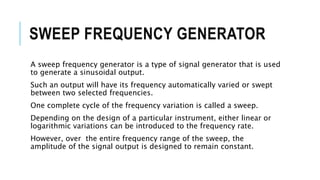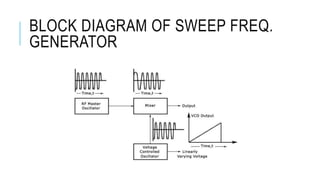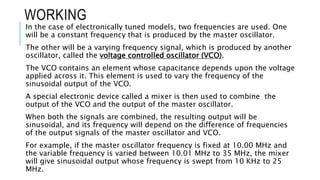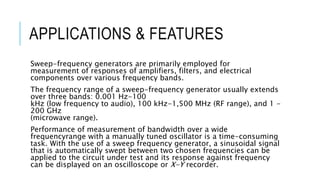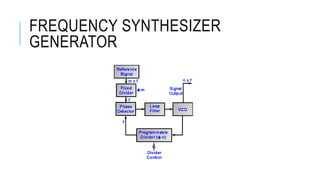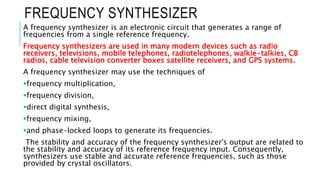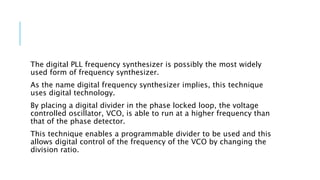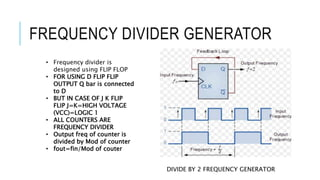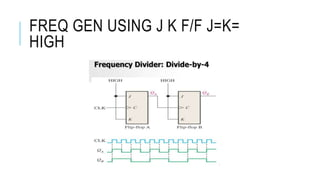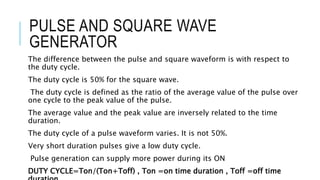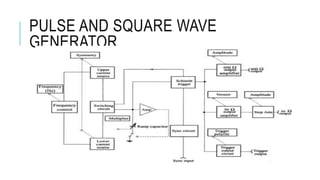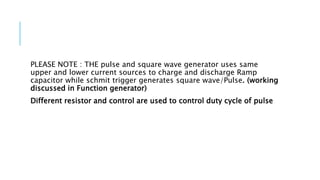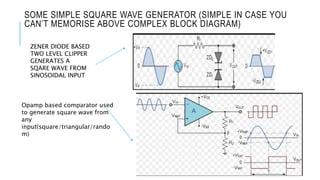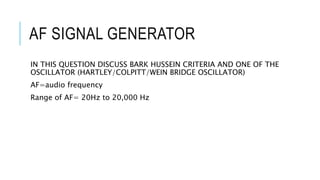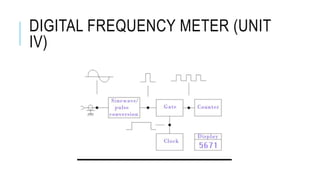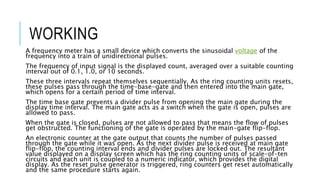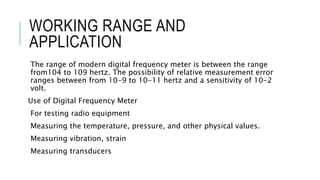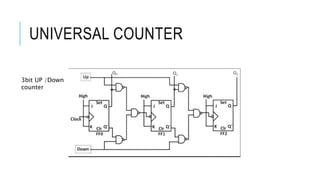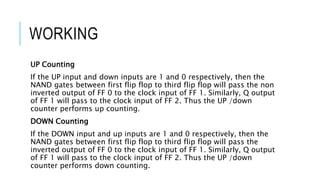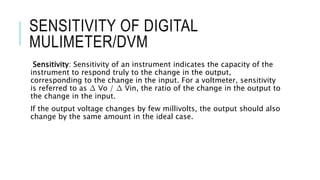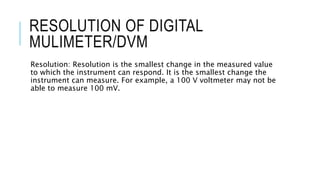1 de 22

### Unit 3 betl 305

• 2. SWEEP FREQUENCY GENERATOR A sweep frequency generator is a type of signal generator that is used to generate a sinusoidal output. Such an output will have its frequency automatically varied or swept between two selected frequencies. One complete cycle of the frequency variation is called a sweep. Depending on the design of a particular instrument, either linear or logarithmic variations can be introduced to the frequency rate. However, over the entire frequency range of the sweep, the amplitude of the signal output is designed to remain constant.
• 3. BLOCK DIAGRAM OF SWEEP FREQ. GENERATOR
• 4. WORKING In the case of electronically tuned models, two frequencies are used. One will be a constant frequency that is produced by the master oscillator. The other will be a varying frequency signal, which is produced by another oscillator, called the voltage controlled oscillator (VCO). The VCO contains an element whose capacitance depends upon the voltage applied across it. This element is used to vary the frequency of the sinusoidal output of the VCO. A special electronic device called a mixer is then used to combine the output of the VCO and the output of the master oscillator. When both the signals are combined, the resulting output will be sinusoidal, and its frequency will depend on the difference of frequencies of the output signals of the master oscillator and VCO. For example, if the master oscillator frequency is fixed at 10.00 MHz and the variable frequency is varied between 10.01 MHz to 35 MHz, the mixer will give sinusoidal output whose frequency is swept from 10 KHz to 25 MHz.
• 5. APPLICATIONS & FEATURES Sweep-frequency generators are primarily employed for measurement of responses of amplifiers, filters, and electrical components over various frequency bands. The frequency range of a sweep-frequency generator usually extends over three bands: 0.001 Hz-100 kHz (low frequency to audio), 100 kHz-1,500 MHz (RF range), and 1 - 200 GHz (microwave range). Performance of measurement of bandwidth over a wide frequencyrange with a manually tuned oscillator is a time-consuming task. With the use of a sweep frequency generator, a sinusoidal signal that is automatically swept between two chosen frequencies can be applied to the circuit under test and its response against frequency can be displayed on an oscilloscope or X-Y recorder.
• 7. FREQUENCY SYNTHESIZER A frequency synthesizer is an electronic circuit that generates a range of frequencies from a single reference frequency. Frequency synthesizers are used in many modern devices such as radio receivers, televisions, mobile telephones, radiotelephones, walkie-talkies, CB radios, cable television converter boxes satellite receivers, and GPS systems. A frequency synthesizer may use the techniques of frequency multiplication, frequency division, direct digital synthesis, frequency mixing, and phase-locked loops to generate its frequencies. The stability and accuracy of the frequency synthesizer's output are related to the stability and accuracy of its reference frequency input. Consequently, synthesizers use stable and accurate reference frequencies, such as those provided by crystal oscillators.
• 8. The digital PLL frequency synthesizer is possibly the most widely used form of frequency synthesizer. As the name digital frequency synthesizer implies, this technique uses digital technology. By placing a digital divider in the phase locked loop, the voltage controlled oscillator, VCO, is able to run at a higher frequency than that of the phase detector. This technique enables a programmable divider to be used and this allows digital control of the frequency of the VCO by changing the division ratio.
• 9. FREQUENCY DIVIDER GENERATOR • Frequency divider is designed using FLIP FLOP • FOR USING D FLIP FLIP OUTPUT Q bar is connected to D • BUT IN CASE OF J K FLIP FLIP J=K=HIGH VOLTAGE (VCC)=LOGIC 1 • ALL COUNTERS ARE FREQUENCY DIVIDER • Output freq of counter is divided by Mod of counter • fout=fin/Mod of couter DIVIDE BY 2 FREQUENCY GENERATOR
• 10. FREQ GEN USING J K F/F J=K= HIGH
• 11. PULSE AND SQUARE WAVE GENERATOR The difference between the pulse and square waveform is with respect to the duty cycle. The duty cycle is 50% for the square wave. The duty cycle is defined as the ratio of the average value of the pulse over one cycle to the peak value of the pulse. The average value and the peak value are inversely related to the time duration. The duty cycle of a pulse waveform varies. It is not 50%. Very short duration pulses give a low duty cycle. Pulse generation can supply more power during its ON DUTY CYCLE=Ton/(Ton+Toff) , Ton =on time duration , Toff =off time
• 12. PULSE AND SQUARE WAVE GENERATOR
• 13. PLEASE NOTE : THE pulse and square wave generator uses same upper and lower current sources to charge and discharge Ramp capacitor while schmit trigger generates square wave/Pulse. (working discussed in Function generator) Different resistor and control are used to control duty cycle of pulse
• 14. SOME SIMPLE SQUARE WAVE GENERATOR (SIMPLE IN CASE YOU CAN’T MEMORISE ABOVE COMPLEX BLOCK DIAGRAM) ZENER DIODE BASED TWO LEVEL CLIPPER GENERATES A SQARE WAVE FROM SINOSOIDAL INPUT Opamp based comparator used to generate square wave from any input(square/triangular/rando m)
• 15. AF SIGNAL GENERATOR IN THIS QUESTION DISCUSS BARK HUSSEIN CRITERIA AND ONE OF THE OSCILLATOR (HARTLEY/COLPITT/WEIN BRIDGE OSCILLATOR) AF=audio frequency Range of AF= 20Hz to 20,000 Hz
• 17. WORKING A frequency meter has a small device which converts the sinusoidal voltage of the frequency into a train of unidirectional pulses. The frequency of input signal is the displayed count, averaged over a suitable counting interval out of 0.1, 1.0, or 10 seconds. These three intervals repeat themselves sequentially. As the ring counting units resets, these pulses pass through the time-base-gate and then entered into the main gate, which opens for a certain period of time interval. The time base gate prevents a divider pulse from opening the main gate during the display time interval. The main gate acts as a switch when the gate is open, pulses are allowed to pass. When the gate is closed, pulses are not allowed to pass that means the flow of pulses get obstructed. The functioning of the gate is operated by the main-gate flip-flop. An electronic counter at the gate output that counts the number of pulses passed through the gate while it was open. As the next divider pulse is received at main gate flip-flop, the counting interval ends and divider pulses are locked out. The resultant value displayed on a display screen which has the ring counting units of scale-of-ten circuits and each unit is coupled to a numeric indicator, which provides the digital display. As the reset pulse generator is triggered, ring counters get reset automatically and the same procedure starts again.
• 18. WORKING RANGE AND APPLICATION The range of modern digital frequency meter is between the range from104 to 109 hertz. The possibility of relative measurement error ranges between from 10-9 to 10-11 hertz and a sensitivity of 10-2 volt. Use of Digital Frequency Meter For testing radio equipment Measuring the temperature, pressure, and other physical values. Measuring vibration, strain Measuring transducers
• 19. UNIVERSAL COUNTER 3bit UP /Down counter
• 20. WORKING UP Counting If the UP input and down inputs are 1 and 0 respectively, then the NAND gates between first flip flop to third flip flop will pass the non inverted output of FF 0 to the clock input of FF 1. Similarly, Q output of FF 1 will pass to the clock input of FF 2. Thus the UP /down counter performs up counting. DOWN Counting If the DOWN input and up inputs are 1 and 0 respectively, then the NAND gates between first flip flop to third flip flop will pass the inverted output of FF 0 to the clock input of FF 1. Similarly, Q output of FF 1 will pass to the clock input of FF 2. Thus the UP /down counter performs down counting.
• 21. SENSITIVITY OF DIGITAL MULIMETER/DVM Sensitivity: Sensitivity of an instrument indicates the capacity of the instrument to respond truly to the change in the output, corresponding to the change in the input. For a voltmeter, sensitivity is referred to as ∆ Vo / ∆ Vin, the ratio of the change in the output to the change in the input. If the output voltage changes by few millivolts, the output should also change by the same amount in the ideal case.
• 22. RESOLUTION OF DIGITAL MULIMETER/DVM Resolution: Resolution is the smallest change in the measured value to which the instrument can respond. It is the smallest change the instrument can measure. For example, a 100 V voltmeter may not be able to measure 100 mV.